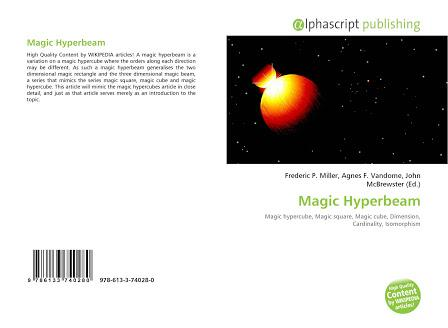# What Do You Know About Magic Hyperbeams?

10 Questions | Total Attempts: 104SettingsOften called n-dimensional magic rectangles, magic hyperbeams are a variation on magic hypercubes that are filled with integers. But unlike magic hypercubes, they have rectangles instead of squares and the orders along each direction of magic hyperbeams may be different. To find out more about magic hyperbeams, there is a short quiz waiting for you.

Related Topics
• 1.
What makes magic hyperbeams different from magic hypercubes?
• A.

The orders along each direction cannot be the same

• B.

The orders along each direction may be different

• C.

It is involved for only circular dimensions

• D.

The orders are in a triangular shape

• 2.
What is the unique feature of magic hyperbeams? ​​​​​
• A.

It generalizes the one dimensional magic rectangle and one dimensional magic beam

• B.

It generalizes the two dimensional magic rectangle and the three dimensional magic beam

• C.

The magic hyperbeam only moves in two dimensions

• D.

The two dimensional magic triangle meets with the three dimension square

• 3.
Which of the following depicts special notation positions in magic hperbeams?
• A.

[ ki; k=[0..n-1]; i=[0..mk-1] ]:

• B.

[ ki; k=[0..n-2]; i=[0..mk-1] ]:

• C.

[ ki; k=[0..n-1]; i=[0..mk+1] ]:

• D.

[ ki; k=[0..n+1]; i=[0..mk-1] ]:

• 4.
When the orders are not relatively prime, what is the n-agonal sum restricted to?
• A.

S = lcm(mi ; i = 0..n-1) (j=0∏n-1mj - 1) / 2

• B.

S = lcm(mi ; i = 0..n-1) (j=0∏n-2mj - 1) / 2

• C.

S = lcm(mi ; i = 0..n-2) (j=0∏n-1mj - 1) / 2

• D.

S = lcm(mi ; i = 0..n-1) (j=0∏n-1mj - 1) / 2

• 5.
What does the term dimension denote in a magic hyperbeam?
• A.

The amount of movements within a hyperbeam

• B.

The amount of orders within a hyperbeam

• C.

The amount of directions within a hyperbeam

• D.

The amount of notations within a hyperbeam

• 6.
When any of the orders m is even in a magic hyperbeam, what does the product become?
• A.

Odd

• B.

Even

• C.

Parallel

• D.

Perpendicular

• 7.
Which letter denotation is used to represent dimensions used in magic hyperbeams?
• A.

A

• B.

H

• C.

N

• D.

L

• 8.
Which of the following gives the formula for basic multiplications in the hyperbeam?
• A.

NB * nB : n[i] = n[ [[i \ m]]∏n-2m] + [i % m]]

• B.

NB * nB : n[i] = n[ [[i \ m]]∏n-3m] + [i % m]]

• C.

NB * nB : n[i] = n[ [[i \ m]]∏n-5m] + [i % m]]

• D.

nB * nB : n[i] = n[ [[i \ m]]∏n-1m] + [i % m]]

• 9.
When dealing with magic hyperbeams, the exception of m=1 allows for general identities. Which of these is an example of such identities?
• A.

Nt = N * N +1

• B.

Nt = N * N -1

• C.

Nt = N * N+2

• D.

Nt = N * N

• 10.
What is the term transpose used to indicate in magic hyperbeams?
• A.

Two dimensional matrices

• B.

Three dimension matrices

• C.

Two dimensional matrices and three dimensional rectangles

• D.

Three dimensional rectangles